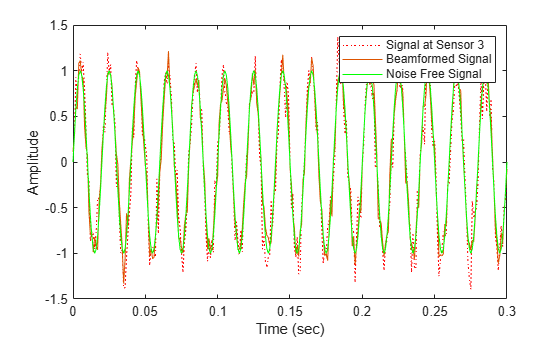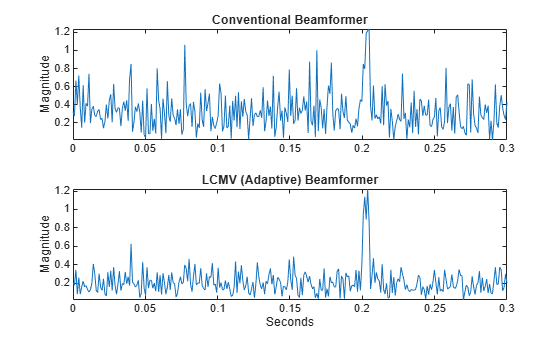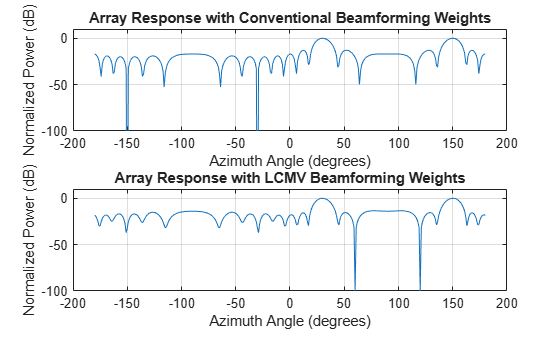phased.LCMVBeamformer

Narrowband LCMV beamformer

Description

The phased.LCMVBeamformer object implements a narrowband linear-constraint minimum-variance (LCMV) beamformer for a sensor array. The LCMV beamformer belongs to the family of constrained optimization beamformers.

To beamform signals arriving at a sensor array:

1. Create the phased.LCMVBeamformer object and set its properties.

2. Call the object with arguments, as if it were a function.

Creation

Description

beamformer = phased.LCMVBeamformer creates an LCMV beamformer System object™, beamformer, with default property values.

beamformer = phased.LCMVBeamformer(Name,Value) creates an LCMV beamformer with each property Name set to a specified Value. You can specify additional name-value pair arguments in any order as (Name1,Value1,...,NameN,ValueN). Enclose each property name in single quotes.

Example: beamformer = phased.LCMVBeamformer('Constraint',[1;1]) sets the constraint matrix.

Properties

expand all

Unless otherwise indicated, properties are nontunable, which means you cannot change their values after calling the object. Objects lock when you call them, and the release function unlocks them.

If a property is tunable, you can change its value at any time.

Constraint matrix, specified as a complex-valued N-by-K matrix. Each column of the matrix represents a constraint. N is the number of elements in the sensor array and K is the number of constraints. K must be less than or equal to N, K ≤ N.

Example: [1 1i;1 1i]

Data Types: single | double
Complex Number Support: Yes

Desired response of the LCMV beamformer, specified as a complex-valued K-by-1 vector, where K is the number of constraints in the Constraint property. Each element in the vector defines the desired response of the constraint specified in the corresponding column of the Constraint property. A value of one creates a distortionless response and a value of zero creates a null response.

Example: [1;0]

Data Types: single | double
Complex Number Support: Yes

Diagonal loading factor, specified as a nonnegative scalar. Diagonal loading is a technique used to achieve robust beamforming performance, especially when the sample size is small. A small sample size can lead to an inaccurate estimate of the covariance matrix. Diagonal loading also provides robustness due to steering vector errors. The diagonal loading technique adds a positive scalar multiple of the identity matrix to the sample covariance matrix.

Tunable: Yes

Data Types: single | double

Enable training data input, specified as false or true. When you set this property to true, use the training data input argument, XT, when running the object. Set this property to false to use the input data, X, as the training data.

Data Types: logical

Enable the output of beamforming weights, specified as false or true. To obtain the beamforming weights, set this property to true and use the corresponding output argument, W. If you do not want to obtain the weights, set this property to false.

Data Types: logical

Usage

Description

example

Y = beamformer(X) performs LCMV beamforming on the input array data, X, and returns the beamformed output in Y.

Y = beamformer(X,XT) uses XT as training data to calculate the beamforming weights. To use this syntax, set the TrainingInputPort property to true.

[Y,W] = beamformer(___) returns the beamforming weights W. To use this syntax, set the WeightsOutputPort property to true.

Input Arguments

expand all

Array element data, specified as anM-by-N matrix where N is the number of elements in the sensor array.

The size of the first dimension of the input matrix can vary to simulate a changing signal length. A size change can occur, for example, in the case of a pulse waveform with variable pulse repetition frequency.

Example: [1 0.5 2.6; 2 -0.2 0]

Data Types: single | double
Complex Number Support: Yes

Training data, specified as a P-by-N matrix. N is the number of elements of the sensor array. P is the length of the training data and must be greater than N.

The size of the first dimension of the input matrix can vary to simulate a changing signal length. A size change can occur, for example, in the case of a pulse waveform with variable pulse repetition frequency.

Example: [1 0.5 2.6; 2 -0.2 0; 3 -2 -1]

Dependencies

To enable this argument, set the TrainingInputPort property to true.

Data Types: single | double
Complex Number Support: Yes

Output Arguments

expand all

Beamformed output, returned as a complex-valued M-by-1 vector.

Data Types: single | double
Complex Number Support: Yes

Beamformer weights, returned as a complex-valued N-by-1 vector. N is the number of elements in the sensor array.

Dependencies

To enable this argument, set the WeightsOutputPort property to true.

Data Types: single | double
Complex Number Support: Yes

Object Functions

To use an object function, specify the System object as the first input argument. For example, to release system resources of a System object named obj, use this syntax:

release(obj)

expand all

 step Run System object algorithm release Release resources and allow changes to System object property values and input characteristics reset Reset internal states of System object

Examples

collapse all

Apply an LCMV beamformer to a 5-element ULA of isotropic sensor elements, preserving the signal from a desired direction. The operating frequency is 300 MHz.

Simulate a low-frequency sinusoid signal in Gaussian noise.

f = 50;
t = (0:.001:.3)';
x = sin(2*pi*f*t);
c = physconst('LightSpeed');
fc = 300e6;
lambda = c/fc;
incidentAngle = [45;0];
antenna = phased.IsotropicAntennaElement('FrequencyRange',[20 20e8]);
array = phased.ULA('NumElements',5,'ElementSpacing',lambda/2,...
'Element',antenna);
x = collectPlaneWave(array,x,incidentAngle,fc,c);
noise = 0.2*(randn(size(x)) + 1j*randn(size(x)));
rx = x + noise;

Beamform the array.

steervec = phased.SteeringVector('SensorArray',array,...
'PropagationSpeed',c);
beamformer = phased.LCMVBeamformer('Constraint',steervec(fc,incidentAngle),'DesiredResponse',1);
y = beamformer(rx);

Plot the original and beamformed signals.

plot(t,real(rx(:,3)),'r:',t,real(y),t,real(x(:,3)),'g')
xlabel('Time (sec)')
ylabel('Amplitude')
legend('Signal at Sensor 3','Beamformed Signal','Noise Free Signal')This example shows how to use an LCMV beamformer to point a null of the array response in the direction of an interfering source. The array is a 10-element uniform linear array (ULA). By default, the ULA elements are isotropic antennas created by the phased.IsotropicAntennaElement System object™. Set the frequency range of the antenna elements so that the carrier frequency lies within the operating range. The carrier frequency is 1 GHz.

fc = 1e9;
lambda = physconst('LightSpeed')/fc;
array = phased.ULA('NumElements',10,'ElementSpacing',lambda/2);
array.Element.FrequencyRange = [8e8 1.2e9];

Simulate a test signal using a simple rectangular pulse.

t = linspace(0,0.3,300)';
testsig = zeros(size(t));
testsig(201:205) = 1;

Assume the rectangular pulse is incident on the ULA from an angle of 30° azimuth and 0° elevation. Use the collectPlaneWave function of the ULA System object to simulate reception of the pulse waveform from the incident angle.

angle_of_arrival = [30;0];
x = collectPlaneWave(array,testsig,angle_of_arrival,fc);

The signal x is a matrix with ten columns. Each column represents the received signal at one of the array elements.

Construct a conventional phase-shift beamformer. Set the WeightsOutputPort property to true to output the spatial filter weights.

convbeamformer = phased.PhaseShiftBeamformer('SensorArray',array,...
'OperatingFrequency',1e9,'Direction',angle_of_arrival,...
'WeightsOutputPort',true);

Add complex-valued white Gaussian noise to the signal x. Set the default random number stream for reproducible results.

rng default
npower = 0.5;
x = x + sqrt(npower/2)*(randn(size(x)) + 1i*randn(size(x)));

Create a 10W interference source. Specify the barrage jammer to have an effective radiated power of 10 W. The interference signal from the barrage jammer is incident on the ULA from an angle of 120° azimuth and 0° elevation. Use the collectPlaneWave function of the ULA System object to simulate reception of the jammer signal.

jamsig = sqrt(10)*randn(300,1);
jammer_angle = [120;0];
jamsig = collectPlaneWave(array,jamsig,jammer_angle,fc);

Add complex-valued white Gaussian noise to simulate noise contributions not directly associated with the jamming signal. Again, set the default random number stream for reproducible results. This noise power is 0 dB below the jammer power. Beamform the signal using a conventional beamformer.

noisePwr = 1e-5;
rng(2008);
noise = sqrt(noisePwr/2)*...
(randn(size(jamsig)) + 1j*randn(size(jamsig)));
jamsig = jamsig + noise;
rxsig = x + jamsig;
[yout,w] = convbeamformer(rxsig);

Implement the adaptive LCMV beamformer using the same ULA array. Use the target-free data, jamsig, as training data. Output the beamformed signal and the beamformer weights.

steeringvector = phased.SteeringVector('SensorArray',array,...
'PropagationSpeed',physconst('LightSpeed'));
LCMVbeamformer = phased.LCMVBeamformer('DesiredResponse',1,...
'TrainingInputPort',true,'WeightsOutputPort',true);
LCMVbeamformer.Constraint = steeringvector(fc,angle_of_arrival);
LCMVbeamformer.DesiredResponse = 1;
[yLCMV,wLCMV] = LCMVbeamformer(rxsig,jamsig);

Plot the conventional beamformer output and the adaptive beamformer output.

subplot(211)
plot(t,abs(yout))
axis tight
title('Conventional Beamformer')
ylabel('Magnitude')
subplot(212)
plot(t,abs(yLCMV))
axis tight
xlabel('Seconds')
ylabel('Magnitude')The adaptive beamformer significantly improves the SNR of the rectangular pulse at 0.2 s.

Using conventional and LCMV weights, plot the responses for each beamformer.

subplot(211)
pattern(array,fc,[-180:180],0,'PropagationSpeed',physconst('LightSpeed'),...
'CoordinateSystem','rectangular','Type','powerdb','Normalize',true,...
'Weights',w)
title('Array Response with Conventional Beamforming Weights');
subplot(212)
pattern(array,fc,[-180:180],0,'PropagationSpeed',physconst('LightSpeed'),...)
'CoordinateSystem','rectangular','Type','powerdb','Normalize',true,...
'Weights',wLCMV)
title('Array Response with LCMV Beamforming Weights');The adaptive beamform places a null at the arrival angle of the interference signal, 120°.

Algorithms

expand all

 Van Trees, H. Optimum Array Processing. New York: Wiley-Interscience, 2002.